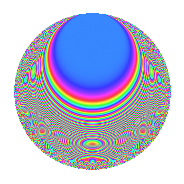# Properties

 Label 175.2.nLevel 175 Weight 2 Character orbit n Rep. character $$\chi_{175}(29,\cdot)$$ Character field $$\Q(\zeta_{10})$$ Dimension 56 Newforms 1 Sturm bound 40 Trace bound 0

# Learn more about

## Defining parameters

 Level: $$N$$ = $$175 = 5^{2} \cdot 7$$ Weight: $$k$$ = $$2$$ Character orbit: $$[\chi]$$ = 175.n (of order $$10$$ and degree $$4$$) Character conductor: $$\operatorname{cond}(\chi)$$ = $$25$$ Character field: $$\Q(\zeta_{10})$$ Newforms: $$1$$ Sturm bound: $$40$$ Trace bound: $$0$$

## Dimensions

The following table gives the dimensions of various subspaces of $$M_{2}(175, [\chi])$$.

Total New Old
Modular forms 88 56 32
Cusp forms 72 56 16
Eisenstein series 16 0 16

## Trace form

 $$56q$$ $$\mathstrut +\mathstrut 12q^{4}$$ $$\mathstrut -\mathstrut 6q^{5}$$ $$\mathstrut +\mathstrut 6q^{9}$$ $$\mathstrut +\mathstrut O(q^{10})$$ $$56q$$ $$\mathstrut +\mathstrut 12q^{4}$$ $$\mathstrut -\mathstrut 6q^{5}$$ $$\mathstrut +\mathstrut 6q^{9}$$ $$\mathstrut -\mathstrut 4q^{10}$$ $$\mathstrut +\mathstrut 8q^{11}$$ $$\mathstrut -\mathstrut 40q^{12}$$ $$\mathstrut -\mathstrut 4q^{14}$$ $$\mathstrut -\mathstrut 18q^{15}$$ $$\mathstrut -\mathstrut 32q^{16}$$ $$\mathstrut +\mathstrut 12q^{19}$$ $$\mathstrut +\mathstrut 12q^{20}$$ $$\mathstrut +\mathstrut 4q^{21}$$ $$\mathstrut -\mathstrut 30q^{22}$$ $$\mathstrut +\mathstrut 10q^{23}$$ $$\mathstrut -\mathstrut 28q^{24}$$ $$\mathstrut +\mathstrut 4q^{25}$$ $$\mathstrut +\mathstrut 12q^{26}$$ $$\mathstrut +\mathstrut 30q^{27}$$ $$\mathstrut -\mathstrut 2q^{29}$$ $$\mathstrut +\mathstrut 28q^{30}$$ $$\mathstrut +\mathstrut 12q^{31}$$ $$\mathstrut +\mathstrut 20q^{33}$$ $$\mathstrut +\mathstrut 2q^{35}$$ $$\mathstrut -\mathstrut 14q^{36}$$ $$\mathstrut -\mathstrut 70q^{37}$$ $$\mathstrut -\mathstrut 70q^{38}$$ $$\mathstrut -\mathstrut 4q^{39}$$ $$\mathstrut -\mathstrut 30q^{40}$$ $$\mathstrut +\mathstrut 4q^{41}$$ $$\mathstrut +\mathstrut 50q^{42}$$ $$\mathstrut +\mathstrut 22q^{44}$$ $$\mathstrut -\mathstrut 52q^{45}$$ $$\mathstrut -\mathstrut 4q^{46}$$ $$\mathstrut -\mathstrut 10q^{47}$$ $$\mathstrut +\mathstrut 30q^{48}$$ $$\mathstrut -\mathstrut 56q^{49}$$ $$\mathstrut -\mathstrut 54q^{50}$$ $$\mathstrut -\mathstrut 44q^{51}$$ $$\mathstrut -\mathstrut 20q^{53}$$ $$\mathstrut +\mathstrut 54q^{54}$$ $$\mathstrut -\mathstrut 2q^{55}$$ $$\mathstrut +\mathstrut 12q^{56}$$ $$\mathstrut +\mathstrut 10q^{58}$$ $$\mathstrut -\mathstrut 6q^{59}$$ $$\mathstrut +\mathstrut 16q^{60}$$ $$\mathstrut -\mathstrut 4q^{61}$$ $$\mathstrut +\mathstrut 50q^{62}$$ $$\mathstrut +\mathstrut 20q^{63}$$ $$\mathstrut +\mathstrut 24q^{64}$$ $$\mathstrut -\mathstrut 18q^{65}$$ $$\mathstrut -\mathstrut 74q^{66}$$ $$\mathstrut +\mathstrut 10q^{67}$$ $$\mathstrut -\mathstrut 78q^{69}$$ $$\mathstrut +\mathstrut 8q^{70}$$ $$\mathstrut -\mathstrut 8q^{71}$$ $$\mathstrut +\mathstrut 140q^{72}$$ $$\mathstrut +\mathstrut 40q^{73}$$ $$\mathstrut +\mathstrut 60q^{74}$$ $$\mathstrut -\mathstrut 8q^{75}$$ $$\mathstrut +\mathstrut 52q^{76}$$ $$\mathstrut -\mathstrut 20q^{77}$$ $$\mathstrut -\mathstrut 90q^{78}$$ $$\mathstrut +\mathstrut 124q^{80}$$ $$\mathstrut -\mathstrut 72q^{81}$$ $$\mathstrut -\mathstrut 30q^{83}$$ $$\mathstrut -\mathstrut 12q^{84}$$ $$\mathstrut +\mathstrut 96q^{85}$$ $$\mathstrut -\mathstrut 20q^{86}$$ $$\mathstrut +\mathstrut 30q^{87}$$ $$\mathstrut +\mathstrut 140q^{88}$$ $$\mathstrut +\mathstrut 38q^{89}$$ $$\mathstrut -\mathstrut 8q^{90}$$ $$\mathstrut +\mathstrut 8q^{91}$$ $$\mathstrut +\mathstrut 80q^{92}$$ $$\mathstrut +\mathstrut 88q^{94}$$ $$\mathstrut -\mathstrut 70q^{95}$$ $$\mathstrut -\mathstrut 28q^{96}$$ $$\mathstrut -\mathstrut 30q^{97}$$ $$\mathstrut +\mathstrut 60q^{99}$$ $$\mathstrut +\mathstrut O(q^{100})$$

## Decomposition of $$S_{2}^{\mathrm{new}}(175, [\chi])$$ into irreducible Hecke orbits

Label Dim. $$A$$ Field CM Traces $q$-expansion
$$a_2$$ $$a_3$$ $$a_5$$ $$a_7$$
175.2.n.a $$56$$ $$1.397$$ None $$0$$ $$0$$ $$-6$$ $$0$$

## Decomposition of $$S_{2}^{\mathrm{old}}(175, [\chi])$$ into lower level spaces

$$S_{2}^{\mathrm{old}}(175, [\chi]) \cong$$ $$S_{2}^{\mathrm{new}}(25, [\chi])$$$$^{\oplus 2}$$